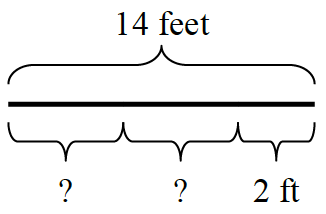### Home > ACC6 > Chapter 8 Unit 8 > Lesson CC1: 8.1.3 > Problem8-41

8-41.

Kayla had a $14$-foot rope that she cut into three pieces. Now two of the pieces are the same length, and the third piece is $2$ feet long.

1. Copy Kayla’s diagram below onto your paper and write an equation that represents the situation. Be sure to remember to define your variable.

Equation: $2x + 2 = 14$
($x$ = unknown length of the first and second pieces)1. Solve your equation and find the length of each of the two equal pieces.

Solve for $x$.